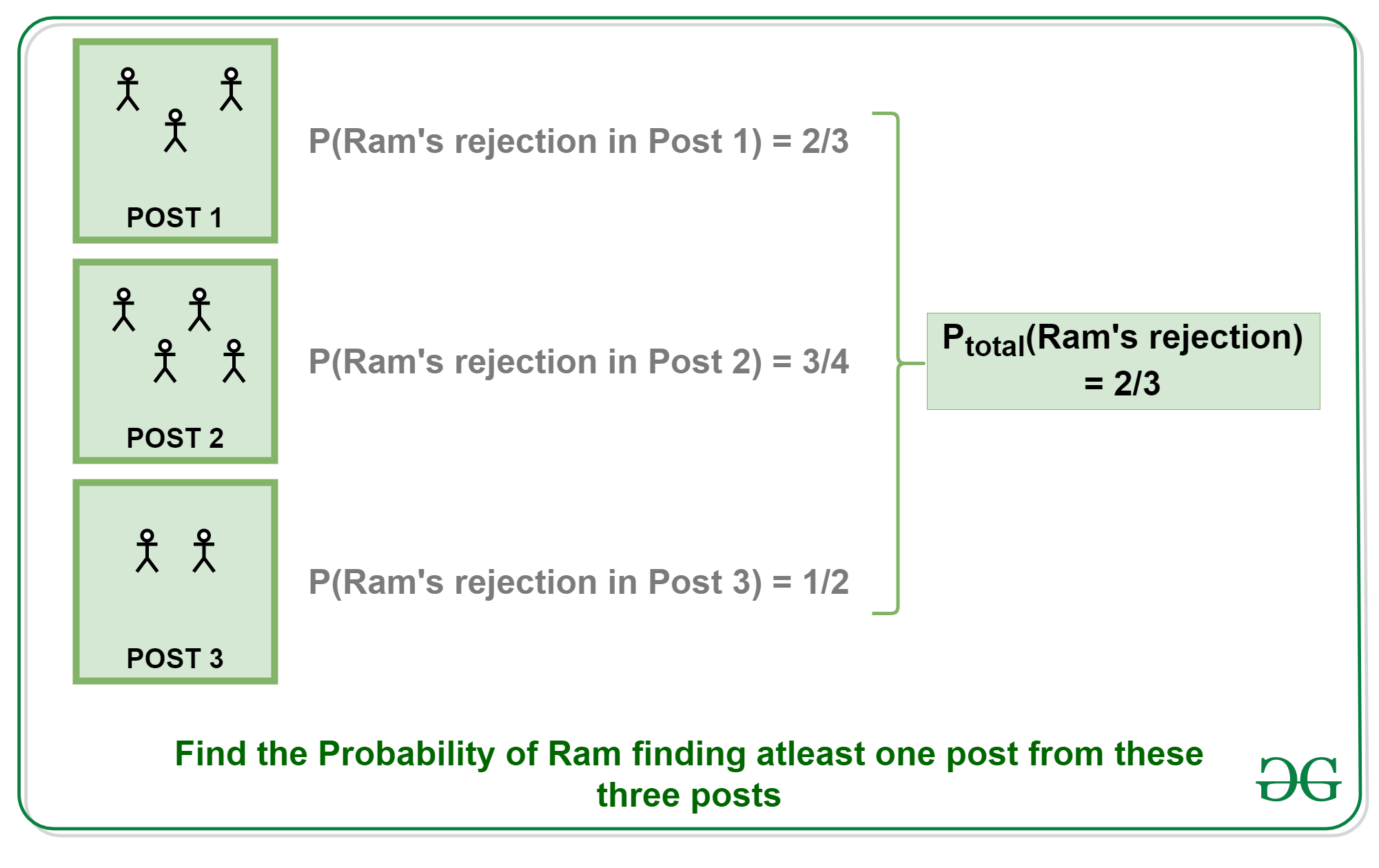# Puzzle | Probability of finding a job

Puzzle: There is a interview going on and Ram is selected for interview to 3 posts. The total number of candidates appearing for the first, second and the third posts are 3, 4 and 2 respectively. Find the probability of Ram getting at least one job.
Assume that the probabilities are independent of each other.

Solution:

1. Since we need to find the probability of Ram getting at least one job, let’s try to find the probability of Ram not getting any jobs.
2. Probability of Ram getting rejected from the interview of the first post is 2/3.
3. Probability of Ram getting rejected from the interview of the second post is 3/4.
4. Probability of Ram getting rejected from the interview of the third post is 1/2.
5.6. Thus the probability of Ram getting rejected from all of the posts = (2/3)*(3/4)*(1/2) = 6/24 = 1/4.
7. Therefore the probability of Ram getting at least one offer = 1-(1/4) = 3/4.

My Personal Notes arrow_drop_upCheck out this Author's contributed articles.

If you like GeeksforGeeks and would like to contribute, you can also write an article using contribute.geeksforgeeks.org or mail your article to contribute@geeksforgeeks.org. See your article appearing on the GeeksforGeeks main page and help other Geeks.

Please Improve this article if you find anything incorrect by clicking on the "Improve Article" button below.

Article Tags :
Practice Tags :

1

Please write to us at contribute@geeksforgeeks.org to report any issue with the above content.